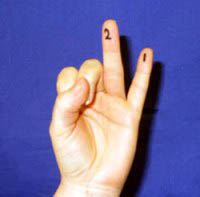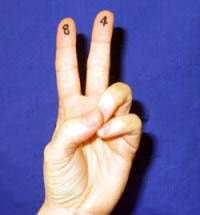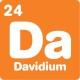What's newDecoding binary numbers

By David, 13 January 2014 Activity

Written by Gabrielle Tramby

In this activity you’ll do maths like a computer. It’s a bit tricky, so younger readers may want to read the ‘What’s Happening?’ section first.

• A pen

What to do

1. Hold up one hand with fingers out-stretched.
2. Starting from left to right, write the numbers 16, 8, 4, 2 and 1 on your fingers. If you are holding up your left hand, your thumb will be number 16 and your little finger will be number one, and the reverse for your right hand.
3. We are going to use these numbers to add up to the number 26.
4. By holding up only your little finger on your left hand you have the number 1. Holding up only your ring finger you have the number two. To get number 3 you need to hold both your little and ring finger as 1 + 2 =3. To get the number twelve you hold up your index and middle fingers and leave the others down, 8 + 4 = 12.
5. Apologise to anyone you made a rude gesture at and tell them maths is always this much fun.Using your left hand, hold up only your little finger, curl down your thumb and the other three fingers. This is the number 1 and is written in binary code as 00001.

How does this relate to binary numbers?

1. Looking at your hand imagine that when your fingers are curled down that fingers represent the value of zero. Standing up right your fingers represent the number one.
2. So if all your fingers are curled down you would write your hand as 00000. If all your fingers are upright it would be 11111.
3. Using your left hand, hold up only your little finger, curl down your thumb and the other three fingers. This is the number 1 and is written in binary code as 00001. We can see this because the first four fingers (including your thumb) are curled down and only the last finger is standing.
4. The number 2 in binary code is 00010 because the ring finger is upright and the others are curled.
5. How would you write the number 12? You would need the finger with the number 8 written on it and the finger with the number 4, 8 + 4 = 12. And therefore, the binary code is 01100 as the second and third fingers are upright. The number 16 is 10000.To get number 3 you need to hold both your little and ring finger as 1 + 2 = 3. This is written in binary code as 00011.

Have a go at coding and decoding

We can code the alphabet by representing letters with numbers. The letter ‘a’ is the number 1, ‘b’ is 2, c = 3, d = 4 and so on until z = 26. This way you can write words as numbers. The word BINARY would be written as 2-9-14-1-18-25.

These numbers can then be coded further using binary numbers. Holding your fingers up again you can work out that the first letter of BINARY, represented by the number 2 can be written as 00010. The second letter (the number 9) is 01001.

Using this method, B-I-N-A-R-Y written in binary code is

00010 – 01001 – 01110 – 0001 – 10010 – 11001For 12, you would need the finger with the number 8 written on it and the finger with the number 4. 8 + 4 = 12. And therefore, the binary code is 01100.

To work out the answer to the question below, first decode the binary into decimal numbers (the numbers will be from 1 to 26) and then decode the decimal numbers into letters.

Question: What’s the difference between pea green paint and the cha cha?

00001- 01110 -11001 – 01111 – 01110 – 00101

00011-00001- 01110

00011-01000- 00001 00011-01000-00001

What’s happening?

When we count and do everyday calculations like adding and multiplying, we use the decimal number system. When computers count, calculate and process words, they use the binary number system.

Using the decimal system, which is based on the number 10, the positions of the digits in a number, reading from the right, mean ‘units’, ‘tens’, ‘hundreds’, ‘thousands’, and so on. (The value of each position goes up by a factor of 10.) The decimal system uses 10 numerals (0, 1, 2, 3, 4, 5, 6, 7, 8, 9) and the number 453 means 3 units, 5 tens and 4 hundreds.

In the binary representation of a number, the position of the digits, mean ‘units’, ‘twos’, ‘fours’, ‘eights’, ‘sixteens’, and so on. The value of each number goes up by a factor of 2. Look at your hand again, did you notice that?

The binary number system uses two numerals (0 and 1) and 1101 means (reading from the right to the left) 1 unit, no twos, 1 four and 1 eight, or 1 + 4 + 8, which equals 13. (A binary digit – a 1 or a 0 – is called a bit.)

Applications

The binary number system is ideal for use in computer programs because the two digits can be represented by the two states of an electronic circuit (off = 0 and on = 1).

Although computers are based on the binary number system, we don’t have to use binary numbers when using one. Instead, we enter decimal numbers the computer converts into binary before manipulating them. Fortunately, computers are much faster than we are at translating decimal into binary.

Binary codes can represent the letters of the alphabet, numerals, common symbols, and commands such as ‘space’ or ‘enter’ on the computer keyboard.

If you’re after more maths activities for kids, subscribe to Double Helix magazine!Categories:

Similar posts

1.What is binary code how do you work it out in homework thank you so much

1.Hi Elishia,
Binary code is a way of writing numbers where you can only use the symbols 1 and 0, and you can’t use 2,3,4,5,6,7,8 or 9.
It’s a bit tricky to explain simply, but instead of having a tens digit and a hundreds digit, you have a twos digit, a fours digit, an eights digit, a sixteens digit and so on.
2.1.